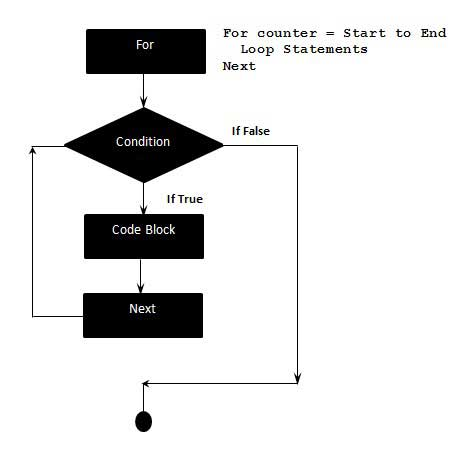# VBScript For Loops

A for loop is a repetition control structure that allows a developer to efficiently write a loop that needs to execute a specific number of times.

## Syntax

The syntax of a for loop in VBScript is −

```For counter = start To end [Step stepcount]
[statement 1]
[statement 2]
....
[statement n]
[Exit For]
[statement 11]
[statement 22]
....
[statement n]
Next
```

## Flow DiagramHere is the flow of control in a For Loop −

• The For step is executed first. This step allows you to initialize any loop control variables and increment the step counter variable.

• Secondly, the condition is evaluated. If it is true, the body of the loop is executed. If it is false, the body of the loop does not execute and flow of control jumps to the next statement just after the For Loop.

• After the body of the for loop executes, the flow of control jumps to the Next statement. This statement allows you to update any loop control variables. It is updated based on the step counter value.

• The condition is now evaluated again. If it is true, the loop executes and the process repeats itself (body of loop, then increment step, and then again condition). After the condition becomes false, the For Loop terminates.

## Example

```<!DOCTYPE html>
<html>
<body>
<script language = "vbscript" type = "text/vbscript">
Dim a : a = 10
For i = 0 to a Step 2 'i is the counter variable and it is incremented by 2
document.write("The value is i is : " & i)
document.write("<br></br>")
Next

</script>
</body>
</html>
```

When the above code is compiled and executed, it produces the following result −

```The value is i is : 0

The value is i is : 2

The value is i is : 4

The value is i is : 6

The value is i is : 8

The value is i is : 10
```
vbscript_loops.htm